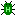#Hemiptera Bugtracker at bugs.linux-forks.de

### Sound of steam engines is played forever

Send replies to 121@bugs.linux-forks.de or using the Form below.From: OP
Sat, 13 Apr 2019 07:26:01 -0000

When I see a running steam engine, its sound is played endlessly and

doesn't fade out when the train is gone. It stops if and only if I

disconnect.

Take a trip by railway 56 with sounds on and then enjoy all the sounds

played endlessy.

If you don't know how to fix it, please, just remove the sound, it's

terrible.From: Someone else
Tue, 16 Apr 2019 15:30:01 -0000

This is one example of an easy to fix bug (remove the sound), which is

there since much longer as 1 yearFrom: Someone else
Mon, 16 Sep 2019 22:15:02 -0000

It seems like this problem can be easily solved without removing the sound:

local function steam_sound_loop(self, dtime, train)

if train.velocity > 0 then -- First make sure that the train isn't standing

if self.sound_loop_handle == nil then -- Make sure that the sound isn't played

self.sound_loop_tmr = 0 -- Start timer

else -- Check if the sound is no longer played

if self.sound_loop_tmr == nil then

self.sound_loop_tmr = 0 -- Add the timer if it doesn't exist

else

self.sound_loop_tmr = self.sound_loop_tmr + dtime -- Add up the timer

if self.sound_loop_tmr >= 5 then -- Make sure that the sound is played for enough time

self.sound_loop_handle = nil -- The sound is no longer played

self.sound_loop_tmr = nil -- Clear the timer

end

end

end

-- If the train is standing, the sound will be stopped in some time. We do not need to interfere with it.

end

end

is_locomotive=true,

drives_on={default=true},

max_speed=10,

seats = {

{

name=S("Driver Stand (left)"),

attach_offset={x=-5, y=10, z=-10},

view_offset={x=9, y=-2, z=-6},

group = "dstand",

},

{

name=S("Driver Stand (right)"),

attach_offset={x=5, y=10, z=-10},

view_offset={x=0, y=6, z=0},

group = "dstand",

},

},

seat_groups = {

dstand={

name = "Driver Stand",

driving_ctrl_access=true,

access_to = {},

},

},

assign_to_seat_group = {"dstand"},

visual_size = {x=1, y=1},

wagon_span=2.05,

collisionbox = {-1.0,-0.5,-1.0, 1.0,2.5,1.0},

custom_on_velocity_change=function(self, velocity)

end

end,

custom_on_step = function(self,dtime,data,train)

steam_sound_loop(self,dtime,train)

end,

custom_on_activate = function(self, staticdata_table, dtime_s)

amount = 10,

time = 0,

-- ^ If time is 0 has infinite lifespan and spawns the amount on a per-second base

minpos = {x=0, y=2.3, z=1.45},

maxpos = {x=0, y=2.3, z=1.4},

minvel = {x=-0.2, y=1.8, z=-0.2},

maxvel = {x=0.2, y=2, z=0.2},

minacc = {x=0, y=-0.1, z=0},

maxacc = {x=0, y=-0.3, z=0},

minexptime = 2,

maxexptime = 4,

minsize = 1,

maxsize = 5,

-- ^ The particle's properties are random values in between the bounds:

-- ^ minpos/maxpos, minvel/maxvel (velocity), minacc/maxacc (acceleration),

-- ^ minsize/maxsize, minexptime/maxexptime (expirationtime)

collisiondetection = true,

-- ^ collisiondetection: if true uses collision detection

vertical = false,

-- ^ vertical: if true faces player using y axis only

texture = "smoke_puff.png",

-- ^ Uses texture (string)

attached = self.object,

})

end,

drops={"default:steelblock 1"},

glow = -1, --supposed to disable effect of light to texture color, so that the entity always appears as full-bright

craft = {recipe = {Status Update